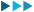Linear Algebra: A Modern Introduction 9781285463247 Algebra & Trigonometry

MRP: 26296
622876%

Thank you for subscribing. You are just one more step away from getting price alerts. Please go to your email inbox & verify your email id.
View more deals from Othersx

Price Graph

1. Current price of Linear Algebra: A Modern Introduction 9781285463247 Algebra & Trigonometry is Rs. 6228
2. Price of Linear Algebra: A Modern Introduction 9781285463247 Algebra & Trigonometry has dropped to its minimum 26 days ago !
3. The lowest price for Linear Algebra: A Modern Introduction 9781285463247 Algebra & Trigonometry was noted on 2019-05-23 04:15:34
4. Linear Algebra: A Modern Introduction 9781285463247 Algebra & Trigonometry is not at it's all time low. You can wait for the price to drop again by setting an alert above. You will be notified as soon as the price drops !
5. Price of Linear Algebra: A Modern Introduction 9781285463247 Algebra & Trigonometry has varied from 3028 to 20044.8 in last 693 days.
6. Percentage Drop shown in the deal is w.r.t MRP of the product. It doesn't depict how good a particular deal is !